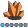qkaiwei
Calcite | Level 5

## How to add moving average after hpfdiagnose

Hi all,

You know, after submitting proc hpfdiagnose, model specifications and model selection list will be generated, now I want to add moving average model to the selection list for each time series,

and what's the way ?

proc hpfarimaspec repository=work.myrep name=mov3;

forecast symbol=y noint p=3

ar=(0.333333333 0.333333333 0.333333333);

estimate noest;

run;

1 ACCEPTED SOLUTION

Accepted Solutionsudo_sas
SAS Employee

## Re: How to add moving average after hpfdiagnose

Hello -

The example below illustrate how to combine model repositories.

Let me know if this helps.

Thanks,

Udo

Proc HPFARIMASPEC MODELREPOSITORY = work.models  SPECNAME=MA_3  SPECLABEL="Moving Average 3 Periods" ;

FORECAST TRANSFORM = NONE  NOINT   P = ( 1 2 3 )  AR = ( 0.3333333333333333 0.3333333333333333 0.3333333333333333 ) ;

ESTIMATE NOEST NOSTABLE;

run;

proc hpfselect modelrep=work.models selectname=my_model;

spec MA_3;

run;

proc hpfdiagnose data=sashelp.air outest=work.myest modelrepository=work.models inselectname=my_model;

forecast air;

id date interval=MONTH acc=total;

arimax;

esm;

run;

proc hpfengine data=sashelp.air inest=work.myest modelrepository=work.models out=_null_ outfor=forecast print=select plot=forecasts;

forecast air;

id date interval=MONTH acc=total;

run;

5 REPLIES 5udo_sas
SAS Employee

## Re: How to add moving average after hpfdiagnose

Hello -

The example below illustrate how to combine model repositories.

Let me know if this helps.

Thanks,

Udo

Proc HPFARIMASPEC MODELREPOSITORY = work.models  SPECNAME=MA_3  SPECLABEL="Moving Average 3 Periods" ;

FORECAST TRANSFORM = NONE  NOINT   P = ( 1 2 3 )  AR = ( 0.3333333333333333 0.3333333333333333 0.3333333333333333 ) ;

ESTIMATE NOEST NOSTABLE;

run;

proc hpfselect modelrep=work.models selectname=my_model;

spec MA_3;

run;

proc hpfdiagnose data=sashelp.air outest=work.myest modelrepository=work.models inselectname=my_model;

forecast air;

id date interval=MONTH acc=total;

arimax;

esm;

run;

proc hpfengine data=sashelp.air inest=work.myest modelrepository=work.models out=_null_ outfor=forecast print=select plot=forecasts;

forecast air;

id date interval=MONTH acc=total;

run;udo_sas
SAS Employee

## Re: How to add moving average after hpfdiagnose

PS: by the way, if you like to run HPFDIAG first, and then append the automatic model repository with your own models, things look a bit different. You will need to "manage" your selection list (in HPFSELECT) yourself; in my first example HPFDIAG will do this for you. Here is an example - note that I had to turn off the seasonality test in order to allow the MA model to be considered in HPFENGINE.

proc hpfdiagnose data=sashelp.air outest=work.myest modelrepository=work.models;

forecast air;

id date interval=MONTH acc=total;

arimax;

esm;

run;

Proc HPFARIMASPEC MODELREPOSITORY = work.models  SPECNAME=MA_3  SPECLABEL="Moving Average 3 Periods" ;

FORECAST TRANSFORM = NONE  NOINT   P = ( 1 2 3 )  AR = ( 0.3333333333333333 0.3333333333333333 0.3333333333333333 ) ;

ESTIMATE NOEST NOSTABLE;

run;

proc hpfselect modelrep=work.models selectname=my_model;

spec MA_3 diag0 diag1;

diagnose seasontest=none;

run;

proc hpfengine data=sashelp.air modelrepository=work.models globalselection=my_model out=_null_ outfor=forecast print=select;

forecast air;

id date interval=MONTH acc=total;

run;qkaiwei
Calcite | Level 5

## Re: How to add moving average after hpfdiagnose

So many thanks!  Solved!qkaiwei
Calcite | Level 5

## Re: How to add moving average after hpfdiagnose

Hi Udo:

One more question. if using combine statment in HPFDiag procedure, It seems that moving average models can't be involved in combination process.

For example, you see, the combine statment only combines valid models from HPFDiag procedure.

Is there an option I can choose to combine all models, whether they are from specification of inSelectName option or from HPFDiag procedure,

Or only do like the following.

Model       Statistic    Selected    Label

diag157     105.25515    No          ARIMA:  sales  ~ D = (1)  Q = 1   NOINT

diag158     139.65636    No          Damped-Trend Exponential Smoothing

diag159      48.72784    No          UCM:  sales  = LEVEL + ERROR

diag160     139.65636    No          COMBINE: AVERAGE(diag157,diag158,diag159)

MA_2         46.81762    Yes         Moving Average 2 Periods

MA_3        125.20871    No          Moving Average 3 Periods

MA_4        166.20584    No          Moving Average 4 Periods

MA_5        180.08417    No          Moving Average 5 Periods

MA_6        208.71758    No          Moving Average 6 Periodsudo_sas
SAS Employee

## Re: How to add moving average after hpfdiagnose

Hello -

When using the automatic combine statement of HPFDIAG only the automatically generated models will be considered.

In order to combine all models you will need to create our own repository and use the combine statement of the HPFSELECT procedure.

See Example 15.8: Using a Combined Forecast of documentation.

Thanks,

Udo

Discussion stats
• 5 replies
• 1380 views
• 3 likes
• 2 in conversation